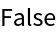#Function Repository Resource:

# IsomorphicHypergraphQ

Determine whether two orderless (undirected) hypergraphs are isomorphic

Contributed by: Jonathan Gorard
 ResourceFunction["IsomorphicHypergraphQ"][h1,h2] yields True if the hypergraphs h1 and h2 are isomorphic, and False otherwise.

## Details and Options

Hypergraphs are specified as lists of hyperedges.
Two hypergraphs are isomorphic if there exists a renaming of vertices that makes them identical.
The algorithm used in ResourceFunction["IsomorphicHypergraphQ"] is a direct generalization of Gorard’s uniqueness tree algorithm for standard graph isomorphism: https://arxiv.org/abs/1606.06399.

## Examples

### Basic Examples (3)

Determine that two hypergraphs are isomorphic:

 In:=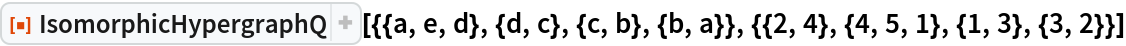Out=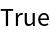Determine that two hypergraphs are not isomorphic:

 In:=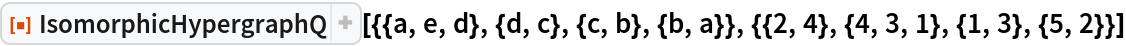Out=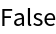Lists of hyperedges that are isomorphic as orderless hypergraphs may not be isomorphic as ordered hypergraphs:

 In:=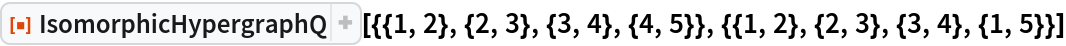Out=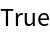### Scope (3)

IsomorphicHypergraphQ also works for standard undirected graphs:

 In:=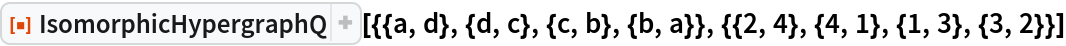Out=In this case, it is functionally equivalent to IsomorphicGraphQ:

 In:=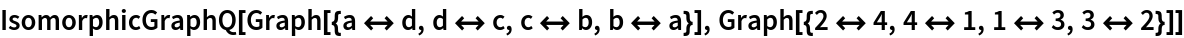Out=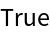Furthermore, IsomorphicHypergraphQ supports isomorphism testing on multihypergraphs (i.e. hypergraphs with multihyperedges):

 In:=Out=